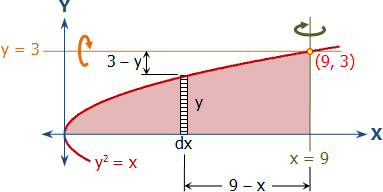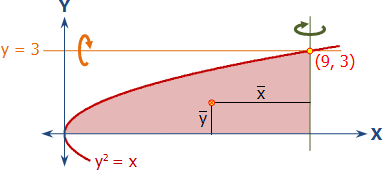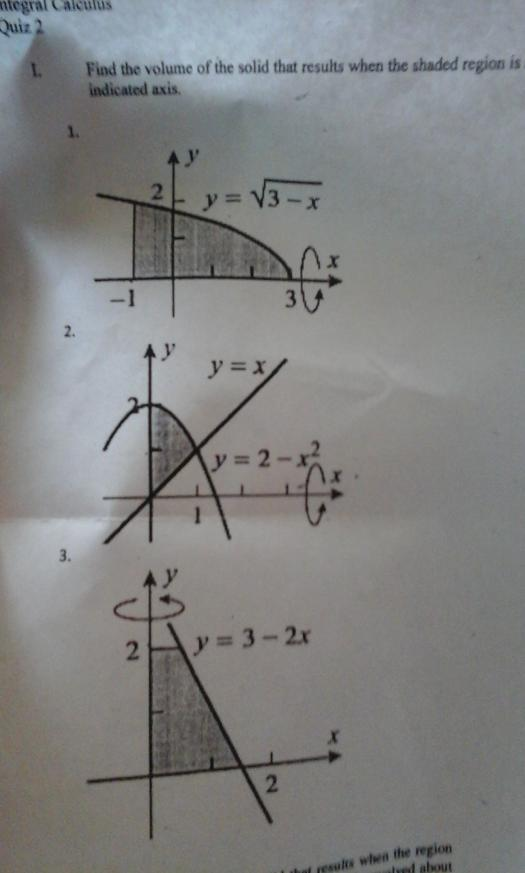# volumes of solids of revolutionproblem no.1
find the volume of the solid that results when the region enclosed by y=squareroot of x,y=0, and x=9 is revolved about the line x=9

prob.no.2
find the volume of the solid that results when the region in problem no. 1 is revolved about the line y=3### Re: volumes of solids of revolution

Volume generated when the area is rotated about the line x = 9

Cylindrical Shell Method
$V = 2\pi \int_a^b} R \, d$

$V = 2\pi \int_{x_1}^{x_2}} (9 - x)(y \, dx$

$V = 2\pi \int_0^9} (9 - x)(\sqrt{x} \, dx$

$V = 129.6\pi ~ \text{unit}^3$Volume generated when the area is rotated about the line y = 3

Circular Disk Method
$V = \pi \int_{x_1}^{x_2}} ({R_{\text{outer}}}^2 - {R_{\text{inner}}}^2) \, d$

$V = \pi \int_0^9} \left[ 3^2 - (3 - y)^2 \right] \, d$

$V = \pi \int_0^9} \left[ 9 - (3 - \sqrt{x})^2 \right] \, d$

$V = 67.5\pi ~ \text{unit}^3$### Re: volumes of solids of revolution

Thank you po### Re: volumes of solids of revolution

Solution by Pappus's Theorem
$A = \frac{2}{3}(3)(9) = 18 ~ \text{unit}^2$

$\bar{x} = \frac{2}{5}(9) = 3.6 ~ \text{units}$

$\bar{y} = \frac{3}{8}(3) = 1.125 ~ \text{units}$Volume generated when the area is rotated about the line x = 9

$V = A \times 2\pi\bar{x}$

$V = 18 \times 2\pi (3.6)$

$V = 129.6\pi ~ \text{unit}^3$

Volume generated when the area is rotated about the line y = 3

$V = A \times 2\pi (3 - \bar{y})$

$V = 18 \times 2\pi (3 - 1.125)$

$V = 67.5\pi ~ \text{unit}^3$### Re: volumes of solids of revolution

pasagot nga po ulit ito..thanks• Mathematics inside the configured delimiters is rendered by MathJax. The default math delimiters are $$...$$ and $...$ for displayed mathematics, and $...$ and $...$ for in-line mathematics.